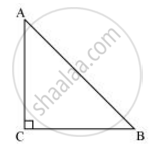Share

# ABC is an isosceles triangle right angled at C. Prove that AB^2 = 2AC^2 - CBSE Class 10 - Mathematics

#### Question

ABC is an isosceles triangle right angled at C. Prove that AB2 = 2AC2

#### SolutionGiven that ΔABC is an isosceles triangle.

∴ AC = CB

Applying Pythagoras theorem in ΔABC (i.e., right-angled at point C), we obtain

AC2 + CB2 = AB2

=> AC2+ AC2 = AB2   (AC = CB)

⇒ 2AC2 = AB2

Is there an error in this question or solution?

#### APPEARS IN

NCERT Solution for Mathematics Textbook for Class 10 (2019 to Current)
Chapter 6: Triangles
Ex. 6.50 | Q: 4 | Page no. 150

#### Video TutorialsVIEW ALL 

Solution ABC is an isosceles triangle right angled at C. Prove that AB^2 = 2AC^2 Concept: Pythagoras Theorem.
S